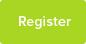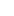Tutor Hunt Questions

A ship P, sails from a point A at noon in a direction of 35 degrees East of North, at 20 knots. One hour later, a second ship Q, sails from A in a direction 80 degrees East of North. At 3p.m. P is due North of Q. Calculate the speed of Q.

The ship P remains at that position, while Q sails on at the same speed and in the same direction for one hour. What is the bearing of Q from P at the end of the hour?

source
Oxford LES GCE O`level winter 1955 Paper I Q10.
ESA Creative Learning
3 years ago

Maths Question asked by David

Please enter your response to the question below. The student will get a notification as soon your response has been approved by our moderation team.

From a point A draw a distance of (20 times 3=) 60 nautical miles (nm) at 35 degrees East of North (start from the North and measure towards the East) for ship P. Draw a second distance of 2x (x times 2, X being the speed of the ship Q) nm at 80 deg for ship Q. Connect Q to P so that it makes the North line (as it is given that P is due North of Q). This gives <PAQ=45 deg (80-35 deg), <QPA=35 deg, <AQP=100 deg (90+10 deg).
Apply Sine rule 2X/Sin35 = 60/Sin100 X = Sin35/2 times 60/Sin100 X = 17.475 knots.
Second Part: Extend the side AQ further from point Q (half as much) to show further distance travelled by ship Q in the extra hour while ship P remains at the previous position. Let’s say Ship Q reaches to a point R. Draw a line to join R to P.
<PAR = <PAQ = 45 deg, AR = 17.5 times 3 = 52.5 nm.
Use Cosin rule to find the distance PR:
Distance PR = square root of {(60) squared + (52.5) squared – 2 (60) (52.5) Cos(45 deg)}
Distance PR = 43.6 nm
Now <QPR = ? <PQR = 80 deg (Q is still sailing 80 deg East of North, ie measured from PQ). QR = 17.5 nm.
Apply Sine rule to triangle PQR:
Sin<QPR/17.5 = Sin 80 deg/43.6 Sin<QPR = Sin 80 deg/43.6 times 17.5 Sin<QPR = 0.395
<QPR = 23.28 deg.
The bearing of Q from P = 180 – 23.28 = 156.72 deg.
I made a mistake. I foolishly misread the question and thought it was 30 knots. New answers

a) 5.82 knots per hour.
b) 156.77 degrees.

1) 17.5 knots
2) 336 degrees
From the information provided, Q is travelling at 5.82 knots. This was done by using the sine rule.
Q would be at the bearing 180-17.5=162.5 degrees 11.58 nautical miles away from P.
17.5 knots
156.76 degrees
Answer (a) 8.74 knots/hour.

Answer (b) 156.73 degrees.

Draw the diagram using accurate bearings then use the "sin" rule to work out how far ship Q has travelled. It took 2 hours to get there, so halve the distance to get the speed.

Extend the diagram and use the "cosine rule" to work out the distance from the new position of Q to P. The use the sin rule again to work out the angle at the top of the new triangle. Then subtract from 180 to get the bearing.

The other way would be to use the accuracy of the diagram and use measure and proportion, but it is difficult to do this to an accurate degree.

Tricky question, with high level thinking skills.
Answered by John | 3 years ago
My first answer should have been 17.5 knots, I made a slight error.
01/01/2016 12:09:49 | comment by John